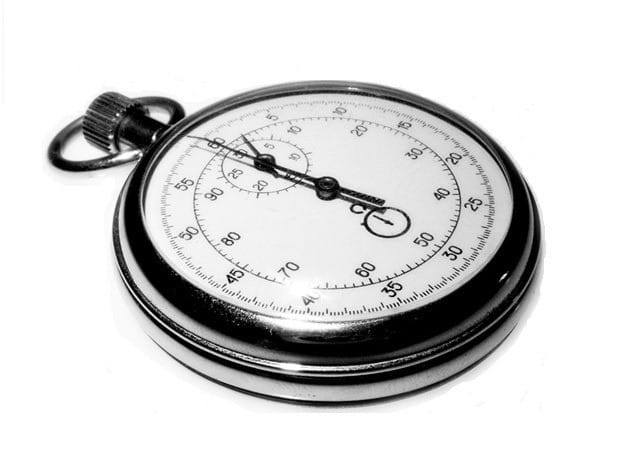﻿

# System.DateTime運算小範例（時間差距、同日判斷）

.NET Framework的System.DateTime類別其實非常的強大，也在所有的專案中被大量地使用。但是有些小地方就算你的程式設計技巧已看過千山萬水，還是有可能會犯錯（或者是繞遠路），因此在這邊分享兩個小技巧。## 計算兩個日期時間物件總共差距多少分鐘（差距幾分鐘）

``````static void Main(string[] args)
{
DateTime t1 = new DateTime(2015, 10, 15, 14, 10, 00);
DateTime t2 = new DateTime(2015, 10, 15, 19, 38, 00);
WriteLine(t2.Subtract(t1).Minutes);  //28
}
``````

``````static void Main(string[] args)
{
DateTime t1 = new DateTime(2015, 10, 15, 14, 10, 00);
DateTime t2 = new DateTime(2015, 10, 15, 19, 38, 00);
WriteLine(new TimeSpan(t2.Ticks - t1.Ticks).TotalMinutes);  //328
}
``````

## 計算兩個日期時間物件是否為同日（同一天）

``````static void Main(string[] args)
{
DateTime t1 = new DateTime(2015, 10, 15, 14, 10, 00);
DateTime t2 = new DateTime(2015, 10, 15, 19, 38, 00);

if (t1.Year == t2.Year &&
t1.Month == t2.Month &&
t1.Day == t2.Day)
{
//BraBra...
}
}
``````

``````static void Main(string[] args)
{
DateTime t1 = new DateTime(2015, 10, 15, 14, 10, 00);
DateTime t2 = new DateTime(2015, 10, 15, 19, 38, 00);

if (DateTime.Compare(t1.Date, t2.Date) == 0)
{
//BraBra...
}
}
``````

System.DateTime運算小範例（迄今時間計算：年月天）